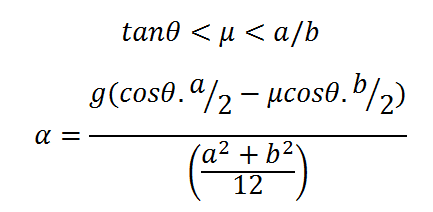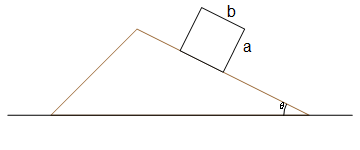# Condition for toppling before sliding of a reactngular block on wedge and Angular acceleration calculator

This CalcTown calculator calculates the condition for toppling of a rectangular block on wedge before it starts sliding and its angular acceleration.

*If µ does not lie between the upper and the lower limits or if the lower limit exceeds the upper limit in magnitude, then the block will slide before it topples. In that case, please ignore the value of angular acceleration.

m
m

#### Result

rad/s2Click here to view image

where

α=Angular acceleration of the block

θ=Angle of the wedge